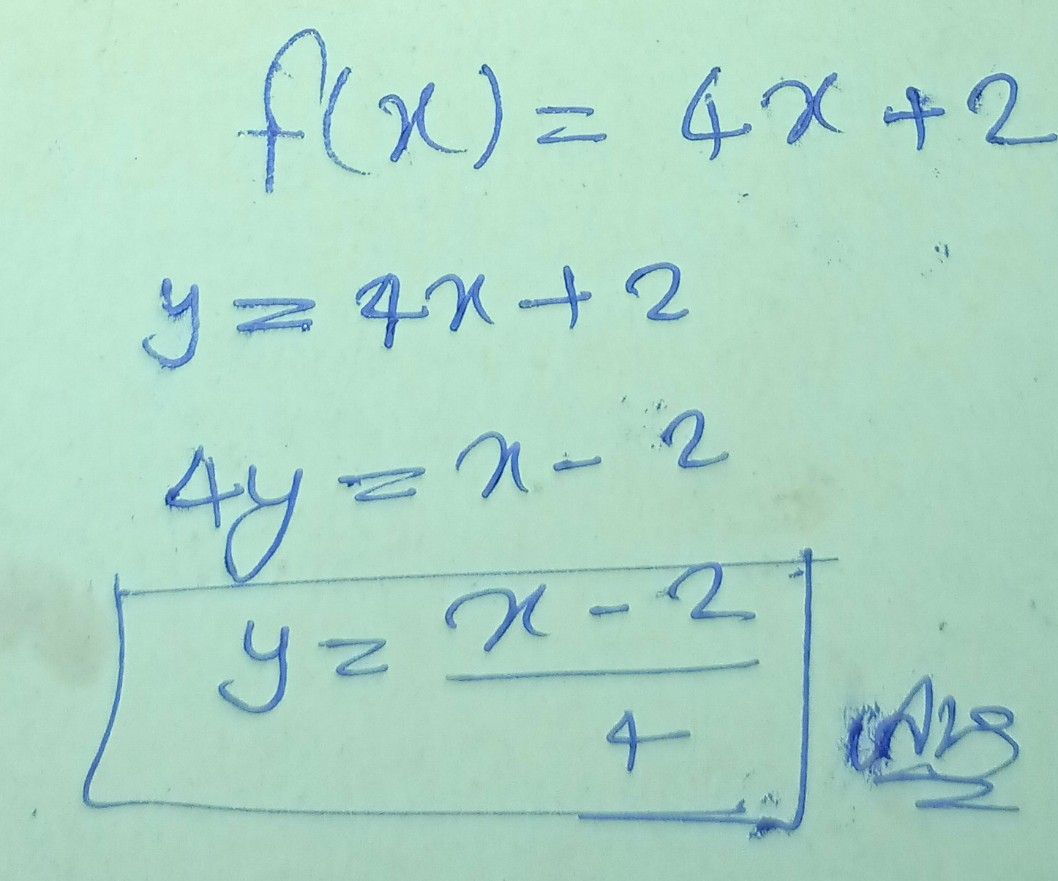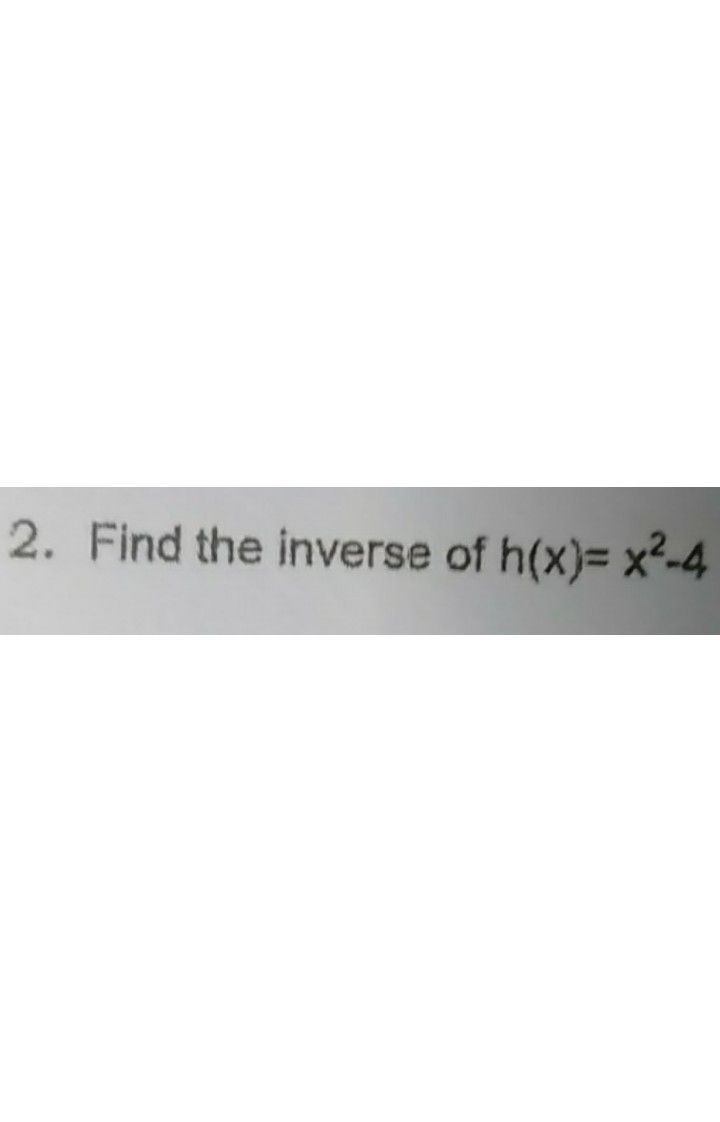Symbol
Problemevery steps. $1.$ Find the inverse of $f\left(x\right)=4x+2$ $2.$ Find the inverse of $h\left(x\right)=x^{2}-4$ $3$ Find the inverse of $g\left(x\right)=\dfrac {x+5} {x-5}$ $4.$ Find the inverse of $f\left(x\right)=\dfrac {-2x+7} {x}$
10th-13th grade
Other
Search count: 111
SolutionQanda teacher - Vandana
check the answerStudent
teacher where the answerQanda teacher - VandanaStudent
thank u teacher
where the #2 answer teacherQanda teacher - Vandana
you can send your other question in preferred match questionStudent